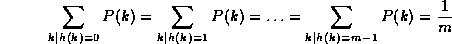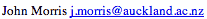Data Structures and Algorithms 8.3.3 Hashing Functions

Choosing a good hashing function, h(k), is essential for hash-table based searching. h should distribute the elements of our collection as uniformly as possible to the "slots" of the hash table. The key criterion is that there should be a minimum number of collisions.

If the probability that a key, k, occurs in our collection is P(k), then if there are m slots in our hash table, a uniform hashing function, h(k), would ensure:Sometimes, this is easy to ensure. For example, if the keys are randomly distributed in (0,r], then,

h(k) = floor((mk)/r)

will provide uniform hashing.

#### Mapping keys to natural numbers

Most hashing functions will first map the keys to some set of natural numbers, say (0,r]. There are many ways to do this, for example if the key is a string of ASCII characters, we can simply add the ASCII representations of the characters mod 255 to produce a number in (0,255) - or we could xor them, or we could add them in pairs mod 216-1, or ...

Having mapped the keys to a set of natural numbers, we then have a number of possibilities.

1. Use a mod function:

h(k) = k mod m.

When using this method, we usually avoid certain values of m. Powers of 2 are usually avoided, for k mod 2b simply selects the b low order bits of k. Unless we know that all the 2b possible values of the lower order bits are equally likely, this will not be a good choice, because some bits of the key are not used in the hash function.

Prime numbers which are close to powers of 2 seem to be generally good choices for m.

For example, if we have 4000 elements, and we have chosen an overflow table organization, but wish to have the probability of collisions quite low, then we might choose m = 4093. (4093 is the largest prime less than 4096 = 212.)

2. Use the multiplication method:

• Multiply the key by a constant A, 0 < A < 1,
• Extract the fractional part of the product,
• Multiply this value by m.

Thus the hash function is:

h(k) = floor(m * (kA - floor(kA)))

In this case, the value of m is not critical and we typically choose a power of 2 so that we can get the following efficient procedure on most digital computers:

• Choose m = 2p.
• Multiply the w bits of k by floor(A * 2w) to obtain a 2w bit product.
• Extract the p most significant bits of the lower half of this product.

It seems that:

A = (sqrt(5)-1)/2 = 0.6180339887

is a good choice (see Knuth, "Sorting and Searching", v. 3 of "The Art of Computer Programming").

3. Use universal hashing:

A malicious adversary can always chose the keys so that they all hash to the same slot, leading to an average O(n) retrieval time. Universal hashing seeks to avoid this by choosing the hashing function randomly from a collection of hash functions (cf Cormen et al, p 229- ). This makes the probability that the hash function will generate poor behaviour small and produces good average performance.

### Key terms

Universal hashing
A technique for choosing a hashing function randomly so as to produce good average performance.

©, 1998